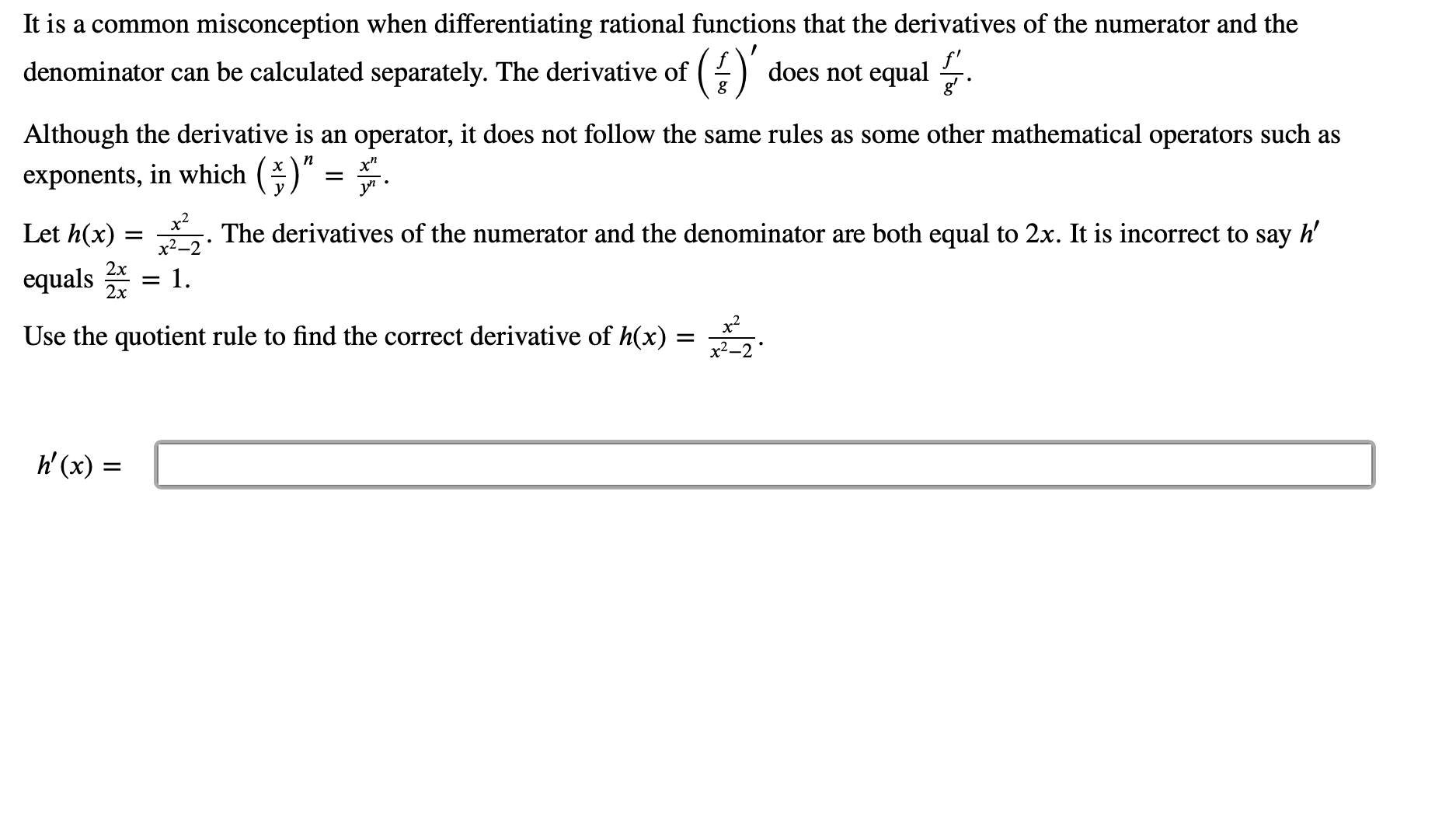# misconception when differentiating rational functions that the derivatives of the numerator and the It is a common () does not equal denominator can be calculated separately. The derivative of Although the derivative is an operator, it does not follow the same rules as some other mathematical operators such as n exponents, in which y х х? Let h(x) h' The derivatives of the numerator and the denominator are both equal to 2x. It is incorrect to say 2x equals - 1 2х x2 Use the quotient rule to find the correct derivative of h(x) х2—2 h(x)=

Questionhelp_outlineImage Transcriptionclosemisconception when differentiating rational functions that the derivatives of the numerator and the It is a common () does not equal denominator can be calculated separately. The derivative of Although the derivative is an operator, it does not follow the same rules as some other mathematical operators such as n exponents, in which y х х? Let h(x) h' The derivatives of the numerator and the denominator are both equal to 2x. It is incorrect to say 2x equals - 1 2х x2 Use the quotient rule to find the correct derivative of h(x) х2—2 h(x)= fullscreen

### Want to see this answer and more?

Experts are waiting 24/7 to provide step-by-step solutions in as fast as 30 minutes!*

*Response times vary by subject and question complexity. Median response time is 34 minutes and may be longer for new subjects.
Tagged in
MathCalculus

### Other